#Function Repository Resource:

# EffectivePrecision

Get the effective precision of an expression

Contributed by: Daniel Lichtblau
 ResourceFunction["EffectivePrecision"][expr] gives the precision of the expression expr, which is a list, equation or similar comprised of numeric subexpressions.

## Details and Options

ResourceFunction["EffectivePrecision"] determines the effective numeric precision of an expression, ignoring heads that are not numeric functions, List, equation or inequality heads, and some related heads.

## Examples

### Basic Examples

A list with an explicit machine number has effective precision of MachinePrecision:

 In:=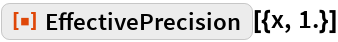Out=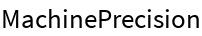If a machine number appears in an expression with a head that is neither a list, equation, inequality nor numeric function, then the effective precision is infinite:

 In:=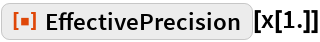Out=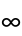### Scope

EffectivePrecision will return the lowest precision encountered in a mathematical subexpression:

 In:=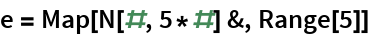Out=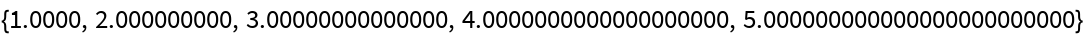In:=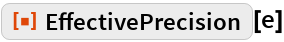Out=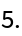MachinePrecision is contagious insofar as it is considered to be lower than any software float precision:

 In:=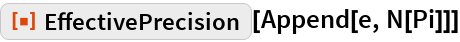Out=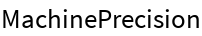### Properties and Relations

EffectivePrecision is intended primarily for mathematical expressions rather than programming language constructs:

 In:=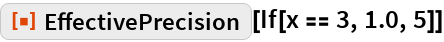Out=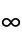To find the lowest precision in an arbitrary expression, use Precision:

 In:=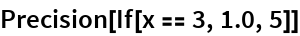Out=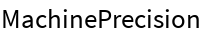## Requirements

Wolfram Language 11.3 (March 2018) or above